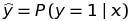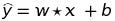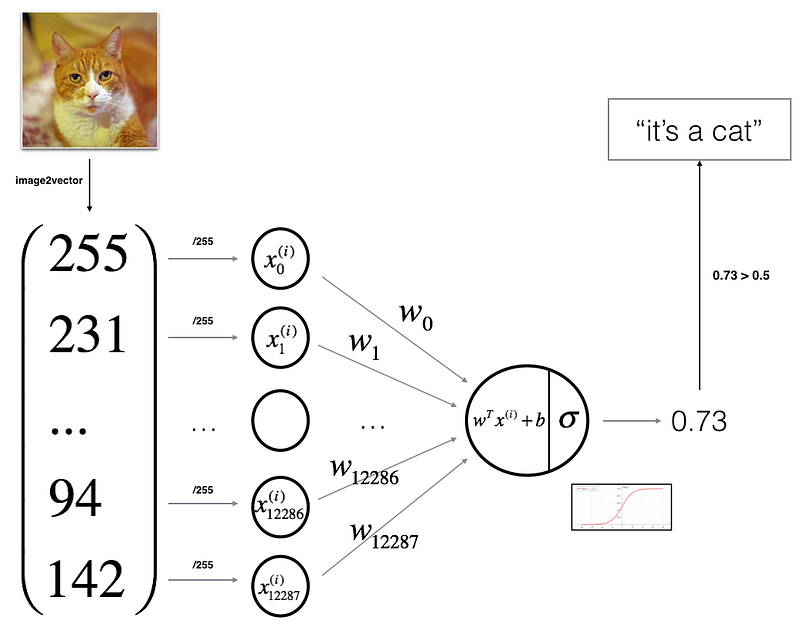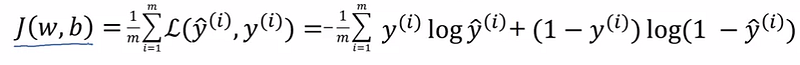# Start NLP with a Single Neuron

### Let's check out a classification problem and explores logistic regression of a neural network as well as who assigns the values of weights and the bias.

· AI Zone · Analysis
Save
3.40K Views

## What Is a Classification Problem?

Classification is an important and central topic in ML, which has to do with training machines how to group together data by particular criteria. Classification is the process where computers group data together based on predetermined characteristics — this is called supervised learning. There is an unsupervised version of classification, called clustering where computers find shared characteristics by which to group data when categories are not specified.

For example:

1. Email Spam: Your goal is to predict whether an email is spam and should be delivered to the Junk folder. (Spam/Not Spam). The text is a simple sequence of words, which is the input (X). The goal is to predict the binary response Y: spam or not.
2. Handwritten Digit Recognition: Your Goal is to identify images of single digits 0–9 correctly. Every entry is an integer ranging from a pixel intensity of 0 (black) to 255 (white). This raw data (X) will be inputted and every image is to be identified as (Y) 0 or 1 or 2 … or 9.

When you take the first problem, it is referred to as Binomial Logistic Regression, where the response variable has two values 0 and 1 or pass and fail or true and false. We can interpret the second problem, but by the means of complexity, I am avoiding the explanation of it. By the way, it is called the multi-class classification problem.

## What Is Logistic Regression?

If you take any neural network, the very basic concept of it will depend on a classification algorithm Logistic Regression. The probability that the output is 1 given its input. It can be represented as the conditional probability:For an example, if the x is an email, then what is the chance that this email is spam?
let’s take input x that has only one value and we want the ŷ. Through the below equation, we derive the ŷ. In this equation w ( called as weights) and b (bias) are the parameters which decide the accuracy of the output ŷ Please note that the ŷ can be any value(100 or 103 or 3002.5 or -50). In order to make it between zero to one, We use a Sigmoid function. It’s not only the function we use, There are many functions which has a different characteristic. For the simplicity of the tutorial, I am not going deeper about it.Normally, the input is not a single value. So, we denote all the inputs as a matrix X. Corresponding values for the parameters as matrix w and b. Take the input x as an n-dimensional matrix. Here, sigma denotes the sigmoid function.

## Let's Go Deeper

From here, you might get the intuition of what happens in a neuron. Look at the below image before going further.It’s a simple diagram that shows the cat prediction by a single neuron. What happens here is that every cat image is transformed to a matrix Vector. For example, an RGB image will contain 3 matrices for Red, Blue, and Green. If we take one matrix as 64*64 pixels, then one matrix will contain 64*64 values. We combine all three vectors into a matrix and input as 1*(64*64*3) matrix. If you take n images, then the matrix will be n*(64*64*3). As shown in the image, the final output will be between zero to one, and we can differentiate the cat image if the value is greater than 0.5.

That’s it!

## Wait…So Who Assigns the Values for Weights and the Bias?

The main part of a neural network is fine-tuning the parameter using the loss function by training the model. We use a loss function to finetune the parameters.

Building a Neural Network model includes these important steps:

1. First, you have to initialize the parameters of the model.
2. Learn the parameters (w,b) for the model by minimizing the cost.
3. Use the learned parameters to make predictions (on the test set).
4. Analyse the results and conclusions.

The below function denotes the total loss for the "m" images. Where ŷ is the value given as the output, and y is the original value. For example, if you take a cat image, ŷ is the output given for the input x by the above logistic regression function, and the 'y' is the actual label where y denotes the label (cat or not) — 0 or 1.## This Doesn’t Make Sense. What Are You Going to Do with These Losses?

Yes, by reducing the loss we increase the accuracy. In order to reduce the loss, the parameters must be updated. If the parameters are updated correctly, then only the output ŷ will automatically be nearer to the y. We use Gradient Decent to make it happen.

“A gradient measures how much the output of a function changes if you change the inputs a little bit.” — Lex Fridman (MIT)